Homework Help Question & Answers

# Y(s) C(s) G(s) R(S) Figure 1: Closed-loop system Q2 Consider the setup in Figure 1 with S s1 (i) ...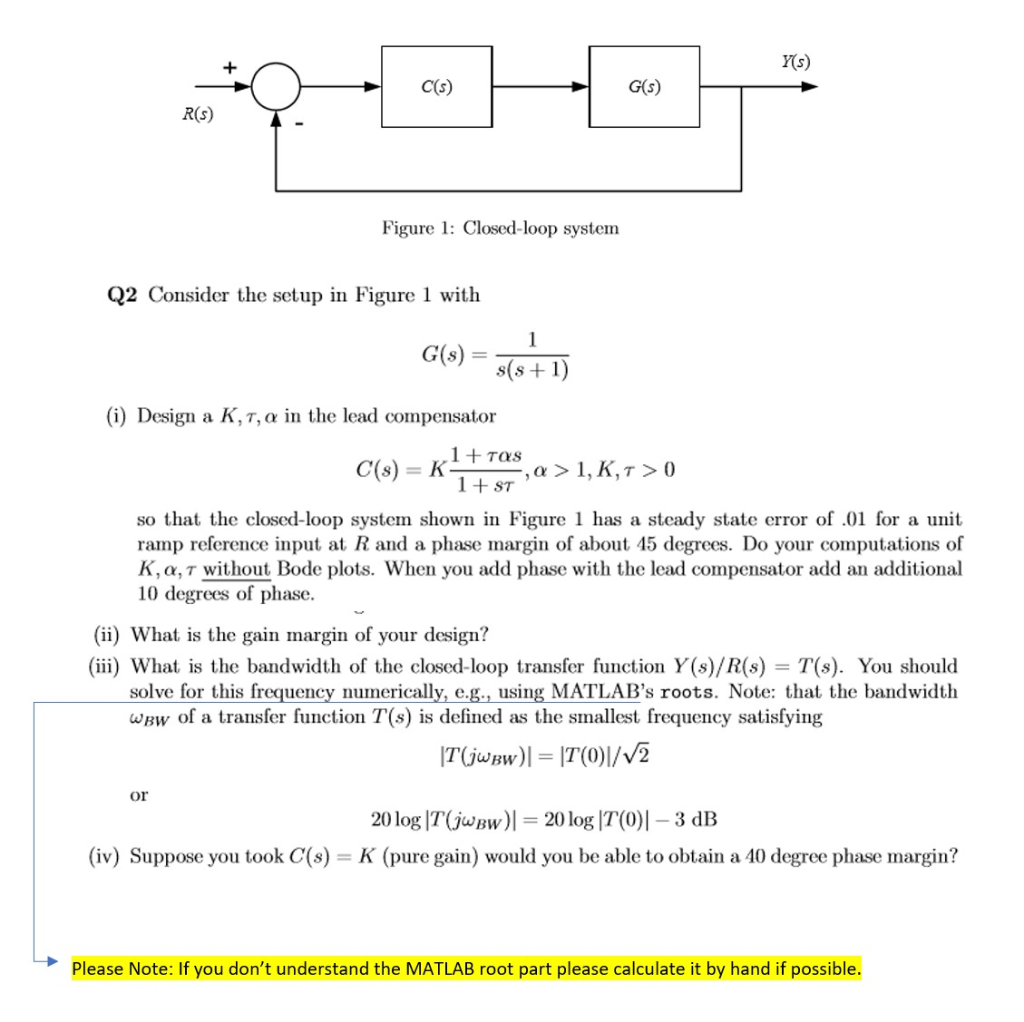Y(s) C(s) G(s) R(S) Figure 1: Closed-loop system Q2 Consider the setup in Figure 1 with S s1 (i) Design a K,τ, α in the lead compensator 1TOS so that the closed-loop system shown in Figure 1 has a steady state error of.0 for a unit ramp reference input at R and a phase margin of about 45 degrees K, α, τ without Bode plots. When you add phase with the lead compensator add an additional 10 degrees of phase. Do your computations of (ii) What is the gain margin of your design? (iii) What is the bandwidth of the closed-loop transfer function Y(s)/R(s) -T(s). You should solve for this frequency numerically, e.g., using MATLAB's roots. Note: that the bandwidth wBw of a transfer function T(s) is defined as the smallest frequency satisfying or 20 log IT(jwBw) K (pure gain) would you be able to obtain a 40 degree phase margin? 20log IT(0)l -3 dB (iv) Suppose you took C(s) Please Note: If you don't understand the MATLAB root part please calculate it by hand if possible

#### Homework Answers

ReportAnswer #1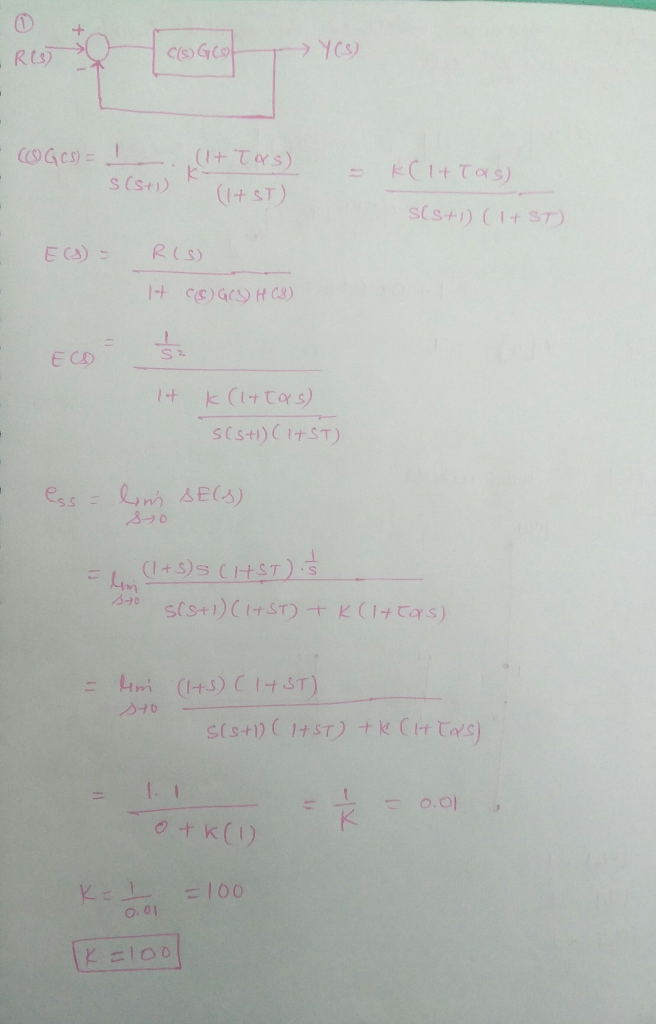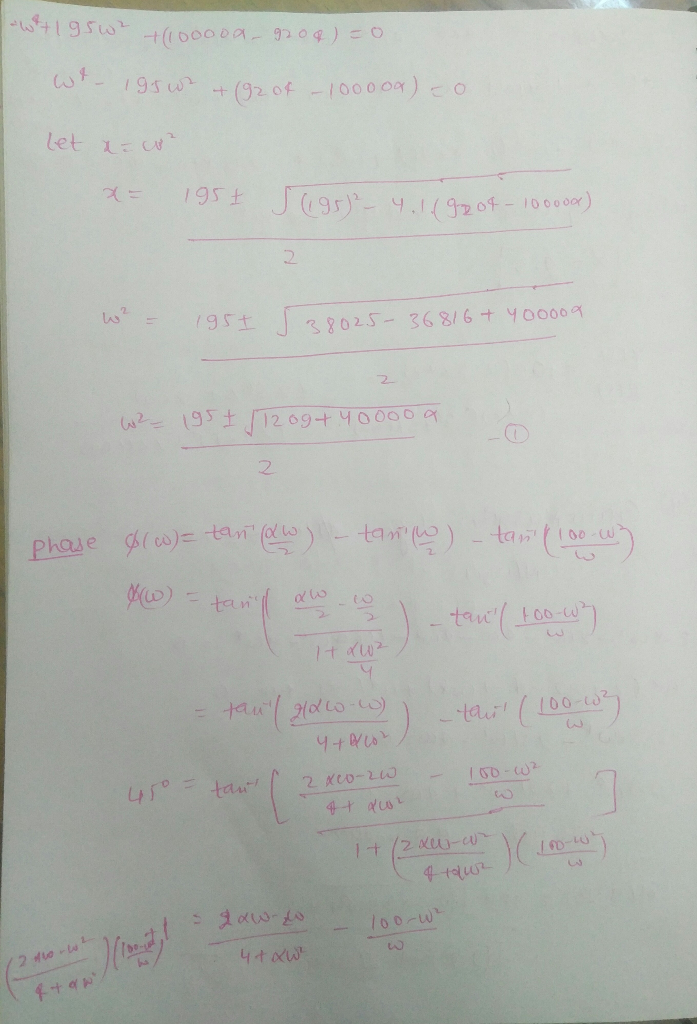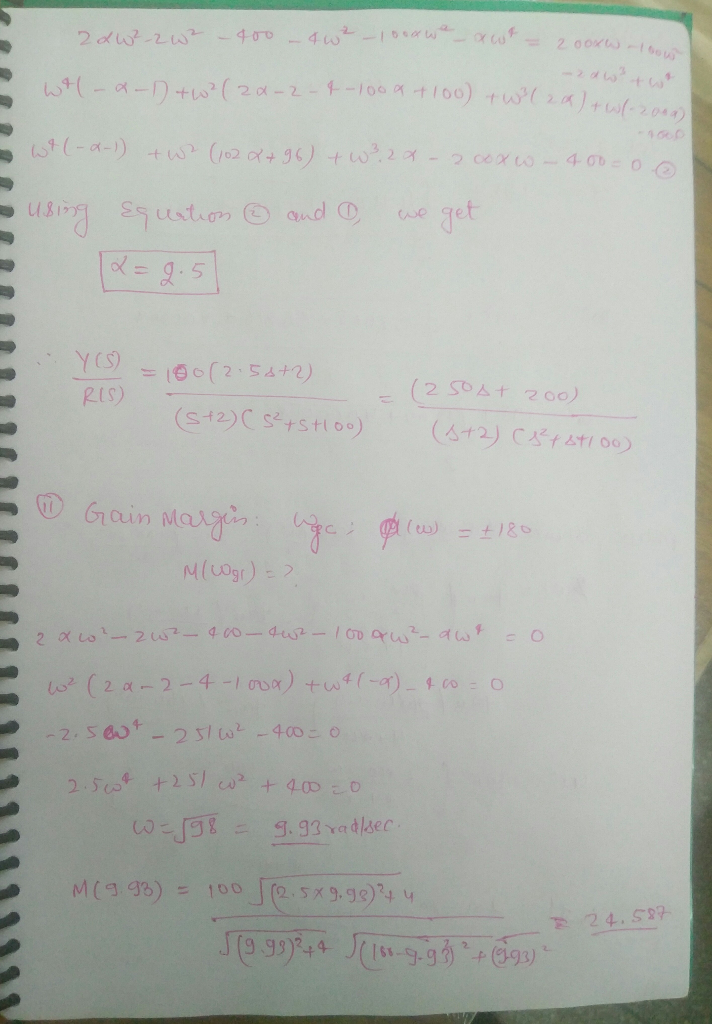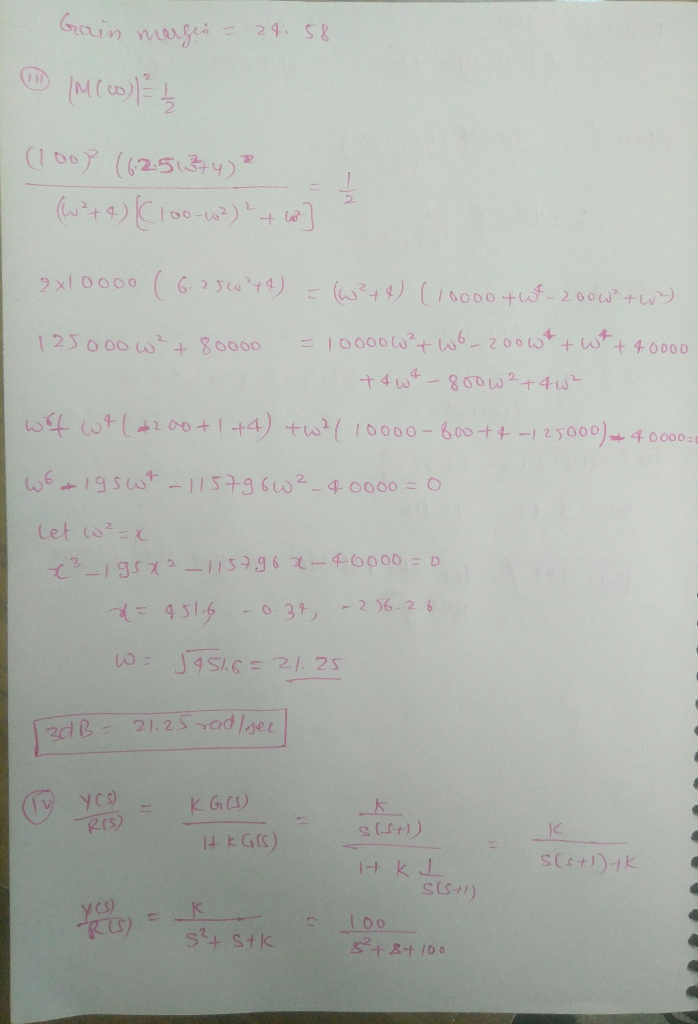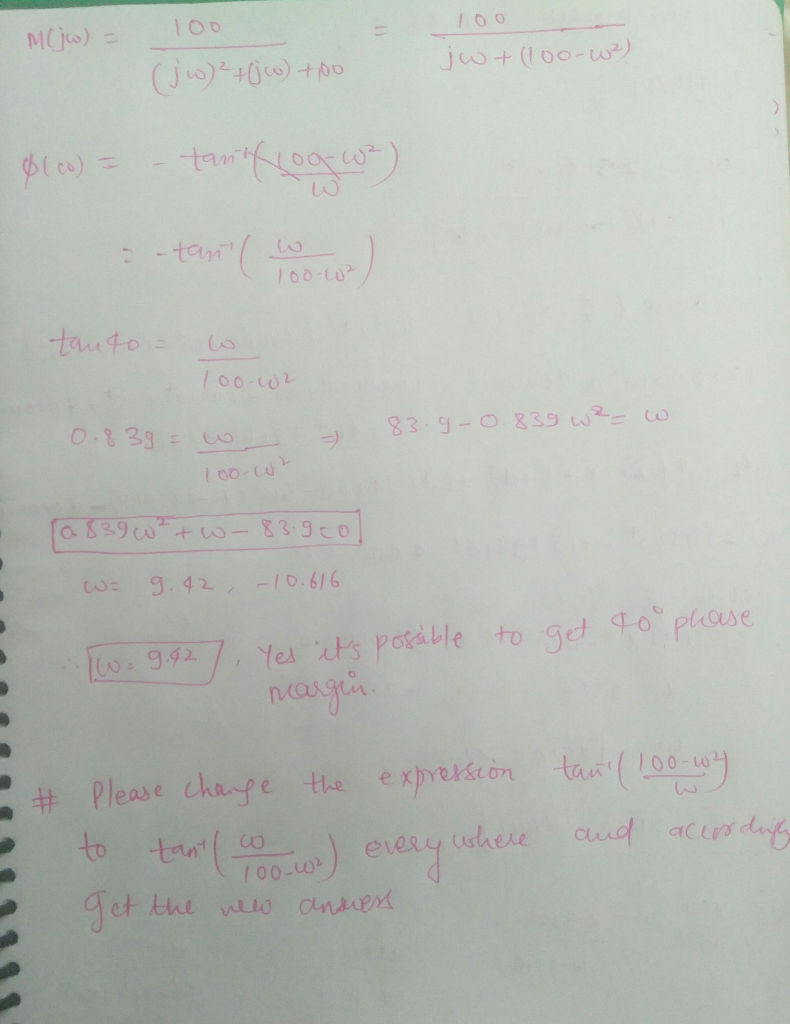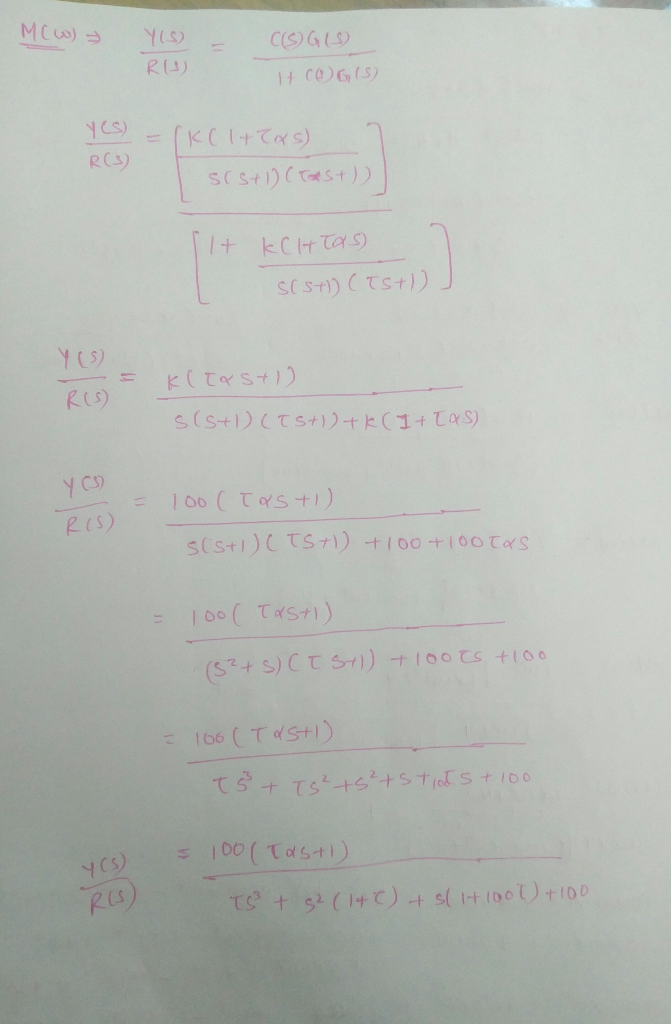Add Homework Help Answer
##### Add Answer of: Y(s) C(s) G(s) R(S) Figure 1: Closed-loop system Q2 Consider the setup in Figure 1 with S s1 (i) ...
Your Answer: Your Name: What's your source?
Not the answer you're looking for? Ask your own homework help question. Our experts will answer your question WITHIN MINUTES for Free.
More Homework Help Questions Additional questions in this topic.

• #### (111) Given a negative unity feedback control system with K(s+α) G(s) = s(s +1)(s+B) Without canceling out any pole/zero, determine the values of α and β such that the closed- loop system will have a...

Need Online Homework Help?

Get FREE EXPERT Answers
WITHIN MINUTES
Related Questions